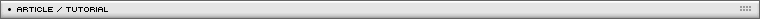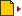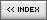3D scripting for Flash: the basics
[ July 22, 2004 ] by Eric Lin
Intermediate 3D scripting for Flash part 1/5:
You will learn the basics on how to setup an interactive 3D camera, with perspective and zoom

Roughly, we know that things in the far distance will appear smaller. So, to make 3D, we can make scale=100/z; That means an ball in 2 m distance will be scale as half, that is scale=50; By this, we can make a beautiful 3D.

```  scale=100/z;
_xscale=_yscale=scale;
_x=x*scale;
_y=y*scale;
```

Compare with common 3d equation : zoom*z0/(z0+z), it is very simillar if we put z0 very small.
Here is the diagram showing why the scale should be zoom*z0/(z0+z);  Or, in the form of M/(N+z); You can adjust M and N to get different effect.
If you like to know more, macromedia has an article telling why and what is the equation.

Well, if we got a camera to take a picture of an object. We get different photo according to several parameters.
1. Usually camera has a zooming option. We can make the picture zoom in and out, but basically the pictures looks the same except the size.
2. A landscape picture will looks different if we take it from far distance or take it from near distance. Definitely it is bigger when we take the picture in the near. However, it is not only the "size", they looks different. It is not just a zoom effect.
3. When we take a picture to an object. It is different whether we are taking it over head or take it from beneath; Of course it would be different if we take it from the left or right. I will explain the Yshift only. It is similar for Xshift.

Here is the 3 constant; zoom, focus, Yshift.

Below is the movie showing the different apperace when 3 constants are changed. The movie contains 6 balls. The x,y are 0 for these 6 balls. Only the z is 0-60-120-180-240-300; So they are aligned along the Z axis.

Here is the code:

```zoomZ = 100;
PZ = {x:150, y:250};
focus = 100;
shiftY=50;

function f3dscale(z) {
var scale = 100*zoomZ/(focus+z);
return scale;
}

function update3dObject(clip) {
with (clip) {
var x3d = x*f3dscale(z)/100+PZ.x;
var y3d = (y+shiftY)*f3dscale(z)/100+PZ.y;
var Y0z0=shiftY*f3dscale(0)/100;
var pt2 = {x:x3d, y:y3d};
_xscale = _root.f3dScale(z);
_yscale = _root.f3dScale(z);
_x = pt2.x;
_y = pt2.y-Y0z0;
swapDepths(10000-z);
}
}
```